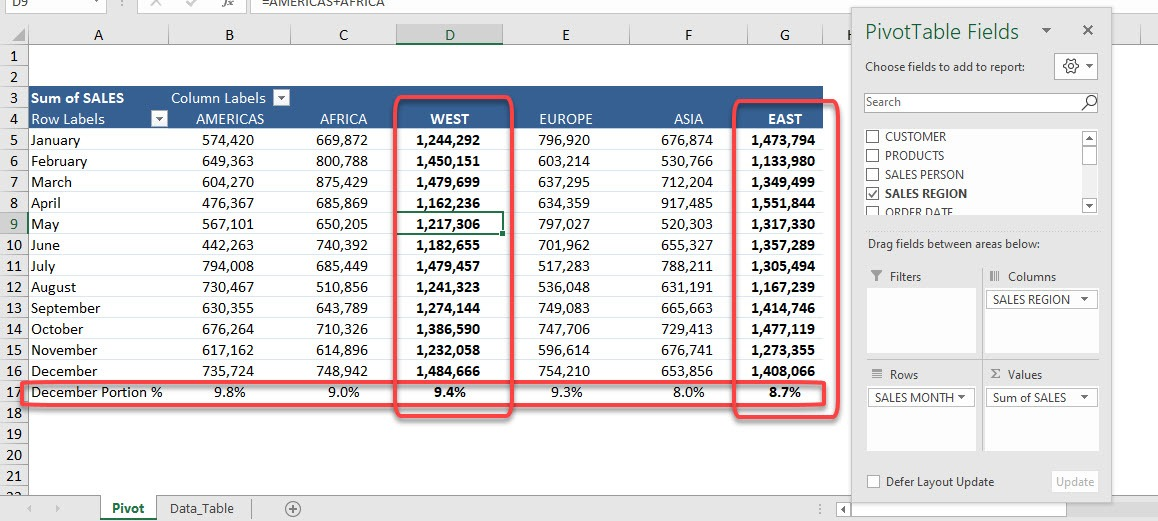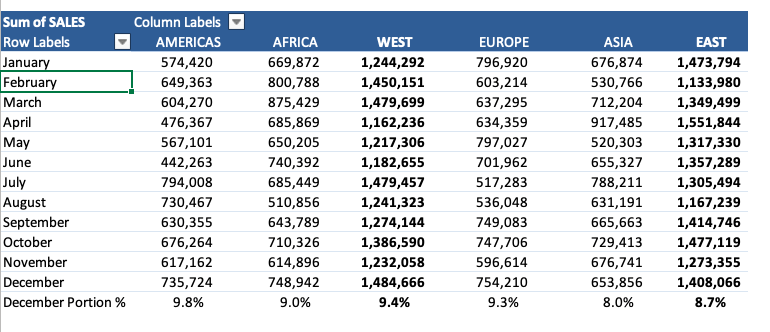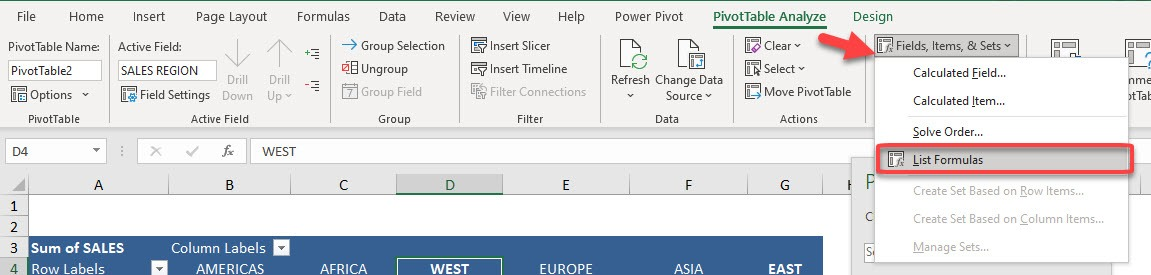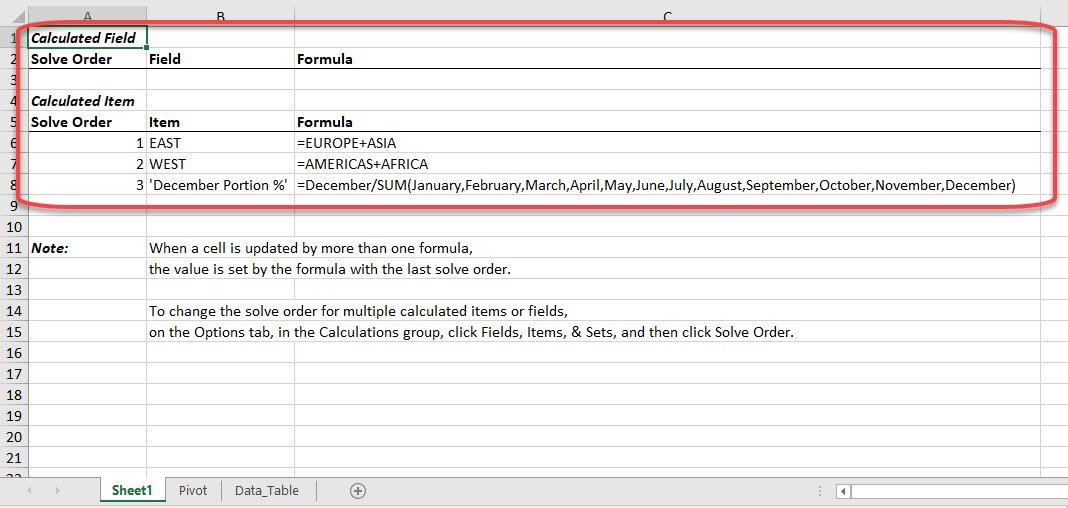Pivot Table Calculated Fields allow you to do mathematical calculations with your Field List and Calculated Items allow you to do mathematical calculations with your Item List. You can use any of the Excel mathematical equations, like /*+-%.

When working with Calculated Fields and Items, it can sometimes get cumbersome to have a quick snapshot of all of them, so that you can quickly check for errors.

Luckily, there is a feature in Excel that can list calculated fields and items for you in one place!

This list will show all the calculated fields and items saved in Pivot Table’s cache, irrespective of whether they are currently being used in the workbook.

Before we proceed, you can download the Exercise Workbook below and follow along with us!

Here is our current Pivot Table and our Calculated Items:Follow the steps below to list calculated fields and items present in the selected Pivot Table in a new worksheet:

STEP 1: Click anywhere on the Pivot Table.STEP 2: Go to PivotTable Analyze > Fields, Items & Sets > List FormulasWith just one click, all of the Calculated Fields and Items are listed in a new worksheet!At first, all the calculated fields will be listed and after that, the calculated items’ list will be shown. You can easily go through all the formulas here and check for errors if any.

You can learn more about Calculated Fields and Calculated Items by reading our blog and watching our YouTube channel.